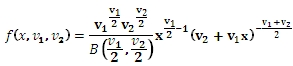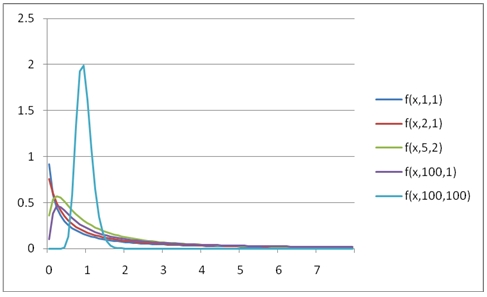# SQL Server F.DIST function

FPDF

Updated: 31 July 2010

Use FPDF to calculate the probability density function of the F distribution.

The probability density function is:Syntax
SELECT [wctStatistics].[wct].[FPDF] (
<@X, float,>
,<@Degrees_freedom1, float,>
,<@Degrees_freedom2, float,>)
Arguments
@X
is any real number. @X is an expression of type float or of a type that implicitly converts to float.
@Degrees_freedom1
is the degrees of freedom. @Degrees_freedom1 is an expression of type float or of a type that implicitly converts to float.
@Degrees_freedom2
is the degrees of freedom. @Degrees_freedom2 is an expression of type float or of a type that implicitly converts to float.
Return Types
float
Remarks
·         @Degrees_freedom1 must be greater than zero (@Degrees_freedom1 > 0).
·         @Degrees_freedom2 must be greater than zero (@Degrees_freedom2 > 0).
·         @X must be greater than or equal to zero (@X ≥ zero).
·          If @X = 0 and @Degrees_freedom1 = 1 and @Degrees_freedom2 =1 then FPDF is equal to infinity
Examples
SELECT wct.FPDF(1,2,1)
This produces the following result
----------------------
0.192450089729876

(1 row(s) affected)

You can use the SeriesFloat function from the XLeratorDB/math library to generate a dataset which can be pasted into EXCEL to generate a graph of the function.
SELECT SeriesValue
,wct.FPDF(SeriesValue, 1, 1) as [f(x,1,1)]
,wct.FPDF(SeriesValue, 2, 1) as [f(x,2,1)]
,wct.FPDF(SeriesValue, 5, 2) as [f(x,5,2)]
,wct.FPDF(SeriesValue, 100, 1) as [f(x,100,1)]
,wct.FPDF(SeriesValue, 100, 100) as [f(x,100,100)]
FROM wct.SeriesFloat(0.1,8,.1,NULL,NULL)

This is an EXCEL-generated graph of the results### SupportCopyright 2008-2021 Westclintech LLC         Privacy Policy        Terms of Service